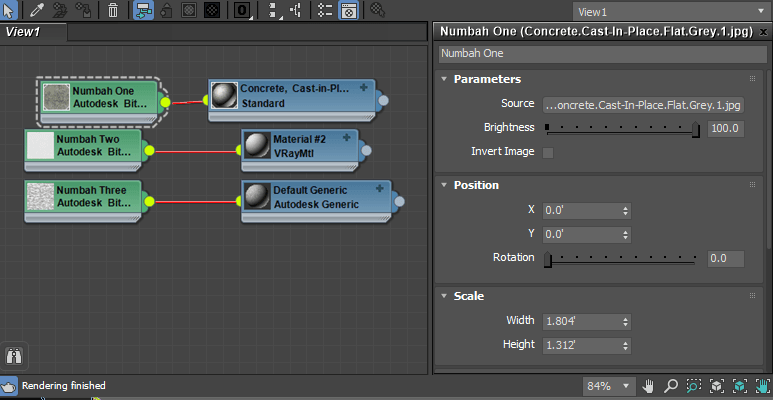# Autodesk_Map to Bitmaptexture

Version:
v1.0
Date Updated:
02/02/2018

This script looks for Autodesk_Map textures in all scene materials and replaces them with standard bitmaps instead. Now that this script is finally general purpose, I'll be rolling a copy of it into the my Autodesk to Standard Material Converter script. The stand-alone version of the script will remain here as usual.

Autodesk_Map textures are generally found in Max files with data imported from Revit.Change Log:

Version 1.0 - 2018.02.02 - Finally found what's been going on here. The script should now work correctly regardless of the material

Version 0.7 - 2014.11.07 - This version now loops through all slots in a Standard material, and keeps most map properties - tiling, offset, etc.

AttachmentSize

## Comment viewing options### Hi fvstudio, I'll test it out

Hi fvstudio, I'll test it out on my end and see if I can figure out what's going on.### How can i use it in max2019？？？？

How can i use it in max2019？？？？### How can i use it in max2019？？？？

Thank you!

Feng### Autodesk_Map and VRay Materials

Finally got around to looking at this one again.

While the script has always worked perfectly for me for converting Autodesk maps that are within Standard materials, users that attempted to use modified versions with VRayMtl would get an error.

What had been going on here was that StandardMaterial has a Maps property, which is an array of all its maps, that I had been relying on for my map replacement. VRayMtl does not have this property, so the script would always fail. Any script that fixed it would have to sample every slot by name.

Fortunately I now have a new method for this, using getClassInstances and Replaceinstances, that bypasses the need to loop through map slots. This not only fixes the script so it works with VRayMtl, but now it should also work for every kind of material regardless of type.### Still not working

Thanks for the reply but this does not work..

-- Script replaces autodesk maps with regular bitmaps. does not handle things like autodesk noise.
-- Reports missing maps

fn convertMap map matname=
(
inMap = map
outMap = bitmaptexture()
print ("converting" + map.name)

outmap.name = inmap.name

outmap.coords.U_Offset = 0
if inMap.Position_X != 0 then outmap.coords.U_Offset = inMap.Position_X
outmap.coords.V_Offset = 0
if inMap.Position_Y != 0 then outmap.coords.V_Offset = inMap.Position_Y

outmap.coords.realWorldScale = on
outmap.coords.U_Tiling = 1
if inMap.Scale_Width != 0 then outmap.coords.U_Tiling = 1/inMap.Scale_Width
outmap.coords.V_Tiling = 1
if inMap.Scale_Height != 0 then outmap.coords.V_Tiling = 1/inMap.Scale_Height

outmap.coordinates.UVW_Type= 0
outmap.coordinates.U_Mirror = false
outmap.coordinates.V_Mirror = false
outmap.coordinates.U_Tile = true
outmap.coordinates.V_Tile = true
outmap.coords.W_angle = inMap.Position_Rotation

try (outmap.bitmap = inmap.Parameters_Source)
catch
(
print ("Map not found in " + inMap.name as string + " in material " + matname + ". Please convert manually.")
)
if badmap == false then (return outMap)
else (return inMap)

)

for m in sceneMaterials do
(
if classof m == VrayMtl then
(
for b=1 to m.maps.count do
(
bmap = m.maps[b]
if isProperty bmap "Parameters_Source" and classof bmap == Autodesk_Map then (m.maps[b] = (convertmap bmap m.name))
)
)
)### Found the problem.

Found the problem. What was going on is that when using classof you shouldn't be putting the parentheses after VRayMtl. so it should look more like this:

for m in sceneMaterials do (if classof m == VRayMtl then print "this is a VRayMtl")### Thanks!

Thanks! Ultimately I want to set it up so it doesn't matter what class of material it is. :)### i do not know why the class

i do not know why the class of m = Vraymtl () does not work### adksbitmap to bitmap for vray materials

-- Script replaces autodesk maps with regular bitmaps. does not handle things like autodesk noise.
-- Reports missing maps

fn convertMap map matname=
(
inMap = map
outMap = bitmaptexture()
print ("converting" + map.name)

outmap.name = inmap.name

outmap.coords.U_Offset = 0
if inMap.Position_X != 0 then outmap.coords.U_Offset = inMap.Position_X
outmap.coords.V_Offset = 0
if inMap.Position_Y != 0 then outmap.coords.V_Offset = inMap.Position_Y

outmap.coords.realWorldScale = on
outmap.coords.U_Tiling = 1
if inMap.Scale_Width != 0 then outmap.coords.U_Tiling = 1/inMap.Scale_Width
outmap.coords.V_Tiling = 1
if inMap.Scale_Height != 0 then outmap.coords.V_Tiling = 1/inMap.Scale_Height

outmap.coordinates.UVW_Type= 0
outmap.coordinates.U_Mirror = false
outmap.coordinates.V_Mirror = false
outmap.coordinates.U_Tile = true
outmap.coordinates.V_Tile = true
outmap.coords.W_angle = inMap.Position_Rotation

try (outmap.bitmap = inmap.Parameters_Source)
catch
(
print ("Map not found in " + inMap.name as string + " in material " + matname + ". Please convert manually.")
)
if badmap == false then (return outMap)
else (return inMap)

)

for m in sceneMaterials do
(
if classof m == VrayMtl () then
(
for b=1 to m.maps.count do
(
bmap = m.maps[b]
if isProperty bmap "Parameters_Source" and classof bmap == Autodesk_Map then (m.maps[b] = (convertmap bmap m.name))
)
)
)